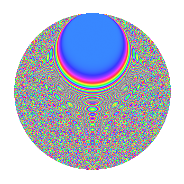# Properties

 Label 1343.2.qLevel 1343 Weight 2 Character orbit q Rep. character $$\chi_{1343}(78,\cdot)$$ Character field $$\Q(\zeta_{16})$$ Dimension 944 Sturm bound 240

# Related objects

## Defining parameters

 Level: $$N$$ = $$1343 = 17 \cdot 79$$ Weight: $$k$$ = $$2$$ Character orbit: $$[\chi]$$ = 1343.q (of order $$16$$ and degree $$8$$) Character conductor: $$\operatorname{cond}(\chi)$$ = $$1343$$ Character field: $$\Q(\zeta_{16})$$ Sturm bound: $$240$$

## Dimensions

The following table gives the dimensions of various subspaces of $$M_{2}(1343, [\chi])$$.

Total New Old
Modular forms 976 976 0
Cusp forms 944 944 0
Eisenstein series 32 32 0

## Trace form

 $$944q - 16q^{2} - 16q^{4} - 16q^{5} - 16q^{8} - 16q^{9} + O(q^{10})$$ $$944q - 16q^{2} - 16q^{4} - 16q^{5} - 16q^{8} - 16q^{9} - 16q^{10} - 16q^{11} + 32q^{18} - 16q^{19} - 16q^{20} - 64q^{21} - 16q^{22} - 32q^{23} - 16q^{25} - 16q^{26} + 48q^{31} + 96q^{32} + 80q^{36} + 64q^{38} + 48q^{40} - 16q^{42} - 144q^{44} - 16q^{45} - 48q^{46} - 80q^{49} - 16q^{51} - 32q^{52} - 80q^{55} - 16q^{62} + 112q^{64} + 16q^{65} - 80q^{72} - 16q^{73} + 112q^{76} + 56q^{79} + 64q^{80} + 80q^{81} + 32q^{83} - 16q^{87} - 16q^{88} - 160q^{89} - 96q^{90} - 16q^{92} - 176q^{95} - 144q^{97} - 16q^{98} - 32q^{99} + O(q^{100})$$

## Decomposition of $$S_{2}^{\mathrm{new}}(1343, [\chi])$$ into newform subspaces

The newforms in this space have not yet been added to the LMFDB.

## Hecke Characteristic Polynomials

There are no characteristic polynomials of Hecke operators in the database